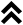# Jobshop explanations

## Input file specifications

There are two input file definitions per instance shown on this page, first the most widely used specification and second the specification used by Taillard . Although both definitions are very similar they are both displayed and downloadable for each instance.

### Standard specification

On the first line are two numbers, the first is the number of jobs and the second the number of machines. Following there is a line for each job. The order for visiting the machines is presented together with the corresponding processing time. The numbering of the machines starts at 0.

For example an instance with only a single job on three machines where the processing time is 5 on machine 1, 6 on machine 2 and 7 on machine 3 and the order that the machines are to be visited by that job is 2,3,1. The instance would be presented as:

 1 3 1 6 2 7 0 5

### Taillard specification

On the first line are two numbers, the first is the number of jobs and the second the number of machines. Following there are two matrice the first with a line for each job containing the processor times for each operation the second with the order for visiting the machines. The numbering of the machines starts at 1.

For example the same instance as above would be presented as:

 1 3 6 7 5 2 3 1

## Operation numbering

The operations are numbered as follows: The first n operations refer to the first operation of each job (according to order of the jobs), operations n+1,...,2n regard the second operation of the n jobs, and so on. So operation i is the k'th operation of job j, where k = ceil(i/n) and j = i mod n.

## Solutions

The solutions are available in several ways. For each solution two images can be found on this site. On each image you can find a hash value which can be used to specify a unique solution, how this hash value can be found is described below. Furthermore, on the largest image the operation order for that solution is depicted.

### Operation order & hash value

For a solution the operations are ordered according to their respective end times in to the non idle schedule of that solution. For operations with equal end times any operation with no duration is always after all operations with a duration, finally as tiebreaker the operation with the lowest machine index comes first. The hash value you find for each solution is the MD5 hash of the operation order seperated by '_', so for solution "2 3 1 4" the string "2_3_1_4" is used to find the hash value b62dced3a30bcc27c69b875a971e18b7 for that solution

### All solutions in a single file

This file consists of a single line per solution. On every line the operations are numbered according to the operation order described above.

### Solution order file

The solution order file gives a line per machine and for each machine the orders of the jobs.

### Solution start file

The solution start file gives a matrix with row per per job and a column per machine, the values in this matrix give the start times for the operations. If the second row starts with a 10, this indicates that the operation of job 2 on machine 1 starts at time 10.

# References

 . Benchmarks for basic scheduling problems.
European Journal of Operational Research, 64.2: 278-285, 1993.